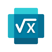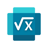# APPOF THEDAYMicrosoft Maths Solver -HW app

Maths Homework Scanner & Tutor

Any calculator can tell you the answer to 437 x 5. But how many can solve an equation like 3x + 5 = 17?

Microsoft Math Solver uses artificial intelligence to solve basic and advanced maths problems – and displays the steps required to figure it all out.Quadratic equations are no match for Microsoft Math Solver.

Simply write out an equation (or use your camera to snap a photo of one) and the app works its magic, even plotting functions on a graph to help you visualise the problem.  For mathletes who want to go deep, the app can search the web for similar problems to work on as well as resources like video lectures about the underlying concepts.